# GED Math : Graphing Lines

## Example Questions

### Example Question #1 : Graphing Lines

A line has slopeand-intercept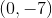. Give its-intercept.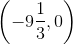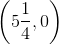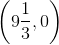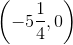Explanation:

The-intercept will be a point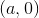for some value. We use the slope formula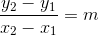,

setting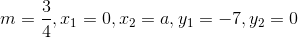,

and solving for: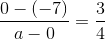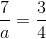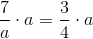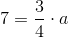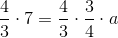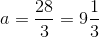The-intercept is.

### Example Question #1 : Graphing Lines

A line has slopeand-intercept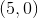. Give its-intercept.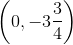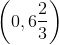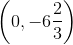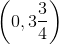Explanation:

The-intercept will be the point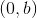for some value. We use the slope formula,

setting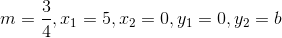,

and solving for: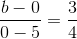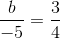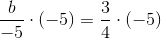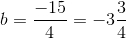The-intercept is.